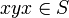# 2-powered twisted subgroup

A subset$S$ of a group$G$ is termed a 2-powered twisted subgroup if it satisfies both the following conditions:
1. For every$x \in S$, there exists a unique element$y \in S$ such that$y^2 = x$.
2.$S$ is a twisted subgroup of$G$, i.e., it contains the identity element, is closed under taking inverses, and for every$x,y \in S$, we have$xyx \in S$.